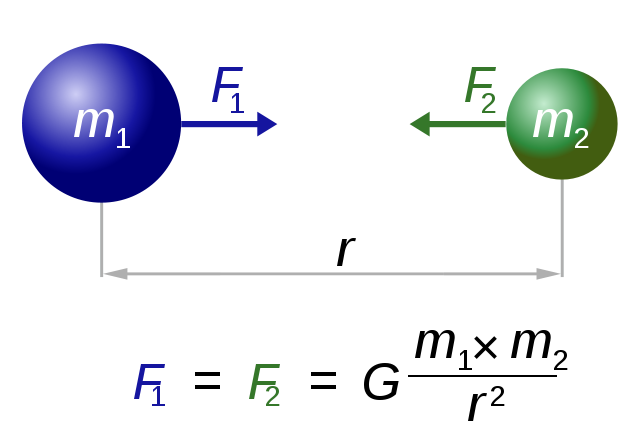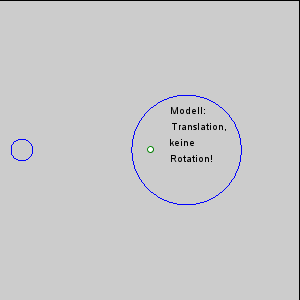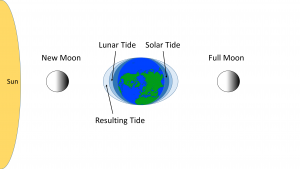# 11.1: Tidal Forces

•• Contributed by Paul Webb
• Professor (Biology) at Roger Williams University

Our modern understanding of tide formation stems from Isaac Newton’sLaw of Universal Gravitation, which states that any two objects have a gravitational attraction to each other. The magnitude of the force is proportional to the masses of the objects, and inversely proportional to the square of the distance between the objects, according to the equation in Figure 11.1.1.Figure 11.1.1 Newton’s Law of Universal Gravitation. The gravitational force between two objects (F) is calculated as the product of the two masses (m1 and m2) divided by the distance between them (r) squared. G is the universal gravitational constant; 6.67408 × 10-11 m3 kg-1 s-2 (By I, Dennis Nilsson [CC BY 3.0], via Wikimedia Commons).

In the case of tides, there are a few other factors that modify this equation so that the distance (r) is cubed rather than squared, giving distance an even greater impact on tidal forces. But for our purposes, the important lesson is that the greater the masses of the objects, the greater the gravitational force, and the farther the objects are from each other, the weaker the force.

Such a gravitational force exists between the Earth and moon, attempting to pull them towards each other. Since the water covering Earth is fluid (unlike the solid land that is more resistant to tidal forces), this gravitational force pulls water towards the moon, creating a “bulge” of water on the side of the Earth facing the moon (Figure 11.1.2). This bulge always faces the moon, while the Earth rotates through it; the regions of Earth moving through the bulge experience a high tide, while those parts of the Earth away from the bulge experience a low tide.Figure 11.1.2 Gravitational forces between the Earth and moon cause a bulge of water to appear on the side of the Earth facing the moon (PW).

If the tides were this simple, everywhere on Earth would see one high tide per day, as there would only be a bulge of water on the side closest to the moon. However, if you have ever looked at tide charts, or lived near the ocean, you probably know that in most places there are two high tides and two low tides per day. Where is this second high tide “bulge” coming from?

The gravitational force between the Earth and moon might be expected to draw the two objects closer together, however, this is not happening. This is because the inward gravitational force is opposed by outward forces that keep the Earth and moon apart. The outward force is an intertial force created by the rotation of the Earth and moon. Contrary to popular belief, the moon is not simply rotating around the Earth; in fact, the Earth and moon are both rotating around each other. Imagine the Earth and moon as equal-sized objects revolving around a point at their center of mass. If both objects had the same mass, the center of rotation would be a point equidistant between the two objects. But since the mass of the Earth is 82 times greater than the mass of the moon, the center of revolution must be closer to the Earth. As an analogy, think about two people on a see-saw. If the people are of roughly equal size, they can sit on either end of the see-saw at it will rotate around a point at equal distance between them. But if the two people have very different masses, such as a large adult and a small child, the larger person must move closer to the pivot point for the see-saw to rotate effectively. In the same way, the center of rotation between the Earth and the moon (the barycenter) must be located closer to the Earth. In fact, the center of rotation lies within the Earth, about 1600 km below the surface. As the Earth and moon rotate around the barycenter, the moon travels much farther than the Earth, giving the impression that the moon is rotating around  Earth (Figure 11.1.3).Figure 11.1.3 Rotation of the Earth and moon around the barycenter (white dot). Because of its larger mass, the center of rotation lies closer to the Earth than to the moon (By Modalanalytiker (Own work) [CC BY-SA 3.0 or GFDL (http://www.gnu.org/copyleft/fdl.html)], via Wikimedia Commons).

The rotation of the Earth-moon system creates an outward inertial force, which balances the gravitational force to keep the two bodies in their orbits. The inertial force has the same magnitude everywhere on Earth, and is always directed away from the moon. Gravitational force, on the other hand, is always directed towards the moon, and is stronger on the side of the Earth closest to the moon. Figure 11.1.4 describes how these forces combine to create the tidal forces. At point O in the center of the Earth, the gravitational force (Fg) and the inertial force (Fr) are equal, and cancel each other out. On the side of Earth closest to the moon, the inward gravitational force (Fg) is greater than the outward inertial force (Fr); the net resulting force (A) is directed towards the moon, and creates a bulge of water on the side facing the moon. On the side of Earth opposite the moon, the outward inertial force is greater than the inward gravitational force; the net resulting force (C) is directed away from the moon, creating a water bulge directed away from the moon.

Now, as the Earth rotates through a 24 hour day, each region passes through two bulges, and experiences two high tides and two low tides per day. This represents Newton’s Equilibrium Theory of Tides, where there are two high tides and two low tides per day, of similar heights, each six hours apart. But as with everything else in oceanography, reality is much more complex than this idealized situation.Figure 11.1.4 Gravitational force (Fg) are strongest closer to the moon and weaker opposite the moon. Inertial forces (Fr) are equal throughout the Earth and directed away from the moon. The tidal forces A and C are the result of the interaction between Fg and Fr and create water bulges on both sides of the Earth, leading to two high tides per day (Vitold Muratov (Own work) [CC BY-SA 3.0], via Wikimedia Commons).

Some of the additional complexity is because in addition to the moon, the sun also exerts tide-affecting forces on Earth. The solar gravitational and inertial forces arise for the same reasons described above for the moon, but the magnitudes of the forces are different. The sun is 27 million times more massive than the moon, but it is 387 times farther away from the Earth. Despite its larger mass, because the sun is so much farther away than the moon, the sun’s gravitational forces are only about half as strong as the moon’s (remember that distance is cubed in the gravity equation). The sun thus creates its own, smaller water bulges, independent of the moon’s, that contribute to the creation of tides.

When the sun, Earth and moon are aligned, as occurs during new and full moons, the solar and lunar bulges are also aligned, and add to each other (constructive interference; see section 10.2) creating an especially high tidal range; high high tides and low low tides (Figure 11.1.5). This period of maximum tidal range is called a spring tide, and they occur every two weeks.Figure 11.1.5 Spring tides with high tidal ranges occur when the solar and lunar tides are added together during full and new moons when the Earth, sun and moon are aligned (PW).

When the sun, Earth and moon are at 90o to each other, the solar and lunar bulges are out of phase, and cancel each other out (destructive interference). Now the tidal range is small, with low high tides and high low tides (Figure 11.1.6). These are neap tides, and occur every two weeks, when the moon is in its 1/4 and 3/4 phases (Figure 11.1.7).Figure 11.1.6 Neap tides are created during 1/4 and 3/4 moons when the Earth, sun and moon are perpendicular to each other. The solar and lunar tides cancel each other out, resulting in a small tidal range (PW).Figure 11.1.7 30 days of tidal data from Bridgeport, CT, USA, showing spring and neap tidal ranges (Modified by PW from Cody Logan (clpo13), Public Domain via Wikimedia Commons).